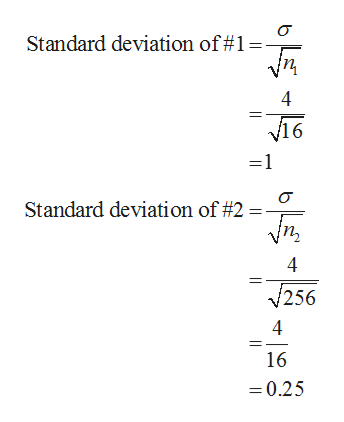A population distribution has a normal shape, with mean μ = 100 and standard deviation σ = 4. Consider two sampling distributions from this population distribution. Sampling distribution #1 is created from the sample means from all possible random samples of size N = 16; sampling distribution #2 is created from the sample means from all possible random samples of size 256. How do the standard deviations compare? The standard deviation of sampling distribution #1 is _____ the standard deviation of sampling distribution #2.

Question

A population distribution has a normal shape, with mean μ = 100 and standard deviation σ = 4. Consider two sampling distributions from this population distribution. Sampling distribution #1 is created from the sample means from all possible random samples of size N = 16; sampling distribution #2 is created from the sample means from all possible random samples of size 256. How do the standard deviations compare? The standard deviation of sampling distribution #1 is _____ the standard deviation of sampling distribution #2.

Step 1

Sampling distribution of sample mean:

If a random sample of size n is taken from a population with mean µ and standard deviation σ, then mean of all sample means is the population mean µ and standard deviation of all sample means is σ/sqrt (n).

Step 2

Comparing the standard deviations:

The population distribution has a normal shape with mean 100 (µ ) and standard deviation 4(σ). First sampling distribution considers all sample means from all possible sample of size 16(...help_outlineImage TranscriptioncloseStandard deviation of#1= 4 v16 =1 Standard deviation of #2 4 256 4 16 =0.25 ьЕ fullscreen

Want to see the full answer?

See Solution

Want to see this answer and more?

Our solutions are written by experts, many with advanced degrees, and available 24/7

See Solution
Tagged in

Other